5.8 Testing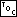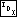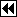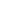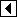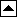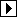Our job isn't done until we can demonstrate that our code works. We'll do this with a test program built using the framework from the previous chapter (see Testing BST Functions). All we have to do is produce functions for AVL trees that correspond to each of those in <bst-test.c 98>. This just involves making small changes to the functions used there. They are presented below without additional comment.

```186. <avl-test.c 186> =
#include <assert.h>
#include <limits.h>
#include <stdio.h>
#include "avl.h"
#include "test.h"

<BST print function; bst => avl 119>
<BST traverser check function; bst => avl 104>
<Compare two AVL trees for structure and content 187>
<Recursively verify AVL tree structure 188>
<AVL tree verify function 190>
<BST test function; bst => avl 100>
<BST overflow test function; bst => avl 122>
```

```187. <Compare two AVL trees for structure and content 187> =
static int compare_trees (struct avl_node *a, struct avl_node *b) {
int okay;

if (a == NULL || b == NULL)     {
assert (a == NULL && b == NULL);
return 1;
}

if (*(int *) a->avl_data != *(int *) b->avl_data
|| ((a->avl_link != NULL) != (b->avl_link != NULL))
|| ((a->avl_link != NULL) != (b->avl_link != NULL))
|| a->avl_balance != b->avl_balance)     {
printf (" Copied nodes differ: a=%d (bal=%d) b=%d (bal=%d) a:",
*(int *) a->avl_data, a->avl_balance,
*(int *) b->avl_data, b->avl_balance);

if (a->avl_link != NULL)         printf ("l");
if (a->avl_link != NULL)         printf ("r");

printf (" b:");
if (b->avl_link != NULL)         printf ("l");
if (b->avl_link != NULL)         printf ("r");

printf ("\n");
return 0;
}

okay = 1;
return okay;
}
```

This code is included in 186.

```188. <Recursively verify AVL tree structure 188> =
/* Examines the binary tree rooted at node.
Zeroes *okay if an error occurs.     Otherwise, does not modify *okay.
Sets *count to the number of nodes in that tree,    including node itself if node != NULL.
Sets *height to the tree's height.
All the nodes in the tree are verified to be at least min    but no greater than max. */
static void recurse_verify_tree (struct avl_node *node, int *okay, size_t *count,
int min, int max, int *height) {
int d;                /* Value of this node's data. */
size_t subcount;   /* Number of nodes in subtrees. */
int subheight;     /* Heights of subtrees. */

if (node == NULL)     {
*count = 0;
*height = 0;
return;
}
d = *(int *) node->avl_data;

<Verify binary search tree ordering 114>

recurse_verify_tree (node->avl_link, okay, &subcount,
min, d -  1, &subheight);
recurse_verify_tree (node->avl_link, okay, &subcount,
d + 1, max, &subheight);
*count = 1 + subcount + subcount;
*height = 1 + (subheight > subheight ? subheight : subheight);

<Verify AVL node balance factor 189>
}
```

This code is included in 186.

```189. <Verify AVL node balance factor 189> =
if (subheight - subheight != node->avl_balance)   {
printf (" Balance factor of node %d is %d, but should be %d.\n",
d, node->avl_balance, subheight - subheight);
*okay = 0;
}
else if (node->avl_balance < -1 || node->avl_balance > +1)   {
printf (" Balance factor of node %d is %d.\n", d, node->avl_balance);
*okay = 0;
}
```

This code is included in 188, 332, 451, and 550.

```190. <AVL tree verify function 190> =
static int verify_tree (struct avl_table *tree, int array[], size_t n) {
int okay = 1;

<Check tree->bst_count is correct; bst => avl 110>

if (okay)     {       <Check AVL tree structure 191>     }

if (okay)     {       <Check that the tree contains all the elements it should; bst => avl 115>     }

if (okay)     {       <Check that forward traversal works; bst => avl 116>     }

if (okay)     {       <Check that backward traversal works; bst => avl 117>     }

if (okay)     {       <Check that traversal from the null element works; bst => avl 118>     }

return okay;
}
```

This code is included in 186, 330, 449, and 548.

```191. <Check AVL tree structure 191> =
/* Recursively verify tree structure. */
size_t count;
int height;

recurse_verify_tree (tree->avl_root, &okay, &count,                      0, INT_MAX, &height);
<Check counted nodes 112>
```

This code is included in 190.5.7 Copying5 AVL Trees 6 Red-Black Trees